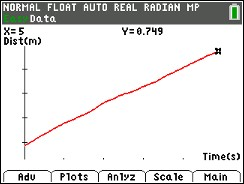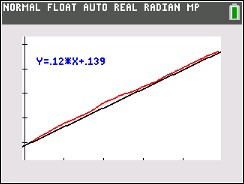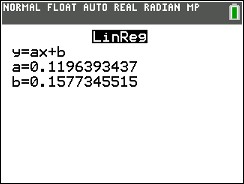••• ##### Device
• TI-83 Plus Family
• TI-84 Plus
• TI-84 Plus Silver Edition
•TI-84 Plus C Silver Edition
•TI-84 Plus CE
•TI-84 Plus CE Python
• ##### Software

TI Connect™
TI Connect™ CE

# Science: Walk the Line

by Texas Instruments#### Overview

In this activity, students' will create constant-speed motion plots and develop linear equations to describe them mathematically.

#### Key Steps

•Distribute the pages to your class.

• Place the CBR on a table and stand 0.5 m away from it
• Walk away from the CBR at a slow and steady pace
• Examine the distance versus time graph
• Record the coordinates and calculate the slope and the starting y- intercept
• Perform a linear regression and compare the slope and the intercept values
•Students create a distance-time graph made by walking at a constant rate in order to find the mathematical equation that duplicates the plot.

•Students use the guess-and-test method to find the equation of the line that matches their data.

•Students use linear regression to gauge the accuracy of their calculations of the slope using the coordinates of two data points.